1594189200

# Cross-Validation — Introduction

In this article, we will go over some of the widely used validation methods employed in machine learning models and their pros and cons. We will see them in action using a real-life dataset in Python.

## General theory

Why do we split the dataset? The simplest answer is to evaluate the performance of the model i.e to determine the predictive power of our model.

The dataset is often split into 2 or 3 parts- train/ test, or train/valid/test set respectively.

The three-part split comes in two flavors :

1. Train/Valid/Test Split

2. Cross-validation with Holdout set

The extra validation set is beneficial for hyperparameters tuning.

In the train/test/split method, the training set is used to tune the hyperparameters. We fit the model in all combinations of the hyperparameter values using the training data. Then, using the fitted model we can predict the validation set and evaluate the performance using all combinations of hyperparameter values. The hyperparameter combination which yields the best results is chosen. The best performing model depends on the evaluation metric — Accuracy, precision, recall we choose. We predict the test set using the hyperparameter combination obtained through the validation set process. The test set is unseen and not used in fitting a model, so it helps evaluate the model performance without any bias.

A drawback of the train/valid/test split method is that there is just ONE validation set to tune the parameters. There is a high risk of “chance” or “luck” in this method of splitting. To overcome this drawback, we can make use of **cross-validation. **This method works similarly to the train/valid/test split method, but we would repeat the splitting steps many times over. There are different types of Cross-validation types- k-fold CV, leave-one-out (LOO), nested CV, etc. Let’s take a look at k-fold CV.

## K-fold CV with holdout set

This is the simplest form of cross-validation.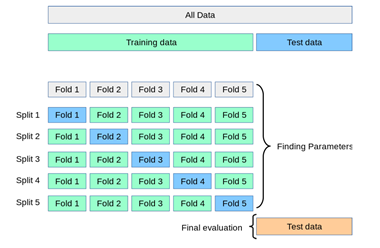This is how the K fold CV works

• A test set is kept aside for final evaluation
• With the remaining data, the data is split into k folds. The model is then trained using the k-1 of the folds (training data). Then, using the kth set (validation set), the prediction is performed using different hyperparameter values and select the hyperparameters that give the best validation score.
• Finally, the model is evaluated with the test set using the best hyperparameters.

# Comparison in Python

We will be using a dataset from the UCI repository. It is a crime rate data from different communities. There are 101 predictor variables such as household size, number of police officers, population, etc. The goal is to predict the total number of non-violate crimes per 100k population (response).

The code and the dataset can be found here.

We will use Lasso regression for our prediction model. Since there are many variables, Lasso is a great tool for filtering out the unnecessary features. With all the other factors being the same, we will implement different validation methods to identify the best hyperparameters for each.

#k-fold #data #machine-learning #crossvalidation #data-science #data analysis

## Buddha Community1594769515

## How to validate mobile phone number in laravel with example

Data validation and sanitization is a very important thing from security point of view for a web application. We can not rely on user’s input. In this article i will let you know how to validate mobile phone number in laravel with some examples.

if we take some user’s information in our application, so usually we take phone number too. And if validation on the mobile number field is not done, a user can put anything in the mobile number field and without genuine phone number, this data would be useless.

Since we know that mobile number can not be an alpha numeric or any alphabates aand also it should be 10 digit number. So here in this examples we will add 10 digit number validation in laravel application.

We will aalso see the uses of regex in the validation of mobile number. So let’s do it with two different way in two examples.

## Example 1:

In this first example we will write phone number validation in HomeController where we will processs user’s data.

``````<?php

namespace App\Http\Controllers;

use Illuminate\Http\Request;
use App\User;

class HomeController extends Controller
{
/**
* Show the application dashboard.
*
* @return \Illuminate\Http\Response
*/
public function create()
{
return view('createUser');
}

/**
* Show the application dashboard.
*
* @return \Illuminate\Http\Response
*/
public function store(Request \$request)
{
\$request->validate([
'name' => 'required',
'phone' => 'required|digits:10',
'email' => 'required|email|unique:users'
]);

\$input = \$request->all();
\$user = User::create(\$input);

return back()->with('success', 'User created successfully.');
}
}
``````

## Example 2:

In this second example, we will use regex for user’s mobile phone number validation before storing user data in our database. Here, we will write the validation in Homecontroller like below.

``````<?php

namespace App\Http\Controllers;

use Illuminate\Http\Request;
use App\User;
use Validator;

class HomeController extends Controller
{
/**
* Show the application dashboard.
*
* @return \Illuminate\Http\Response
*/
public function create()
{
return view('createUser');
}

/**
* Show the application dashboard.
*
* @return \Illuminate\Http\Response
*/
public function store(Request \$request)
{
\$request->validate([
'name' => 'required',
'phone' => 'required|regex:/^([0-9\s\-\+\(\)]*)\$/|min:10',
'email' => 'required|email|unique:users'
]);

\$input = \$request->all();
\$user = User::create(\$input);

return back()->with('success', 'User created successfully.');
}
}
``````

#laravel #laravel phone number validation #laravel phone validation #laravel validation example #mobile phone validation in laravel #phone validation with regex #validate mobile in laravel1666770774

## Variables in Python

In this Python tutorial for beginners, we learn about Variables in Python. Variables are containers for storing data values. A Python variable is a symbolic name that is a reference or pointer to an object.

## Creating Variables

Python has no command for declaring a variable.

A variable is created the moment you first assign a value to it.

### Example

``````x = 5
y = "John"
print(x)
print(y)``````

Variables do not need to be declared with any particular type, and can even change type after they have been set.

### Example

``````x = 4       # x is of type int
x = "Sally" # x is now of type str
print(x)``````

## Casting

If you want to specify the data type of a variable, this can be done with casting.

### Example

``````x = str(3)    # x will be '3'
y = int(3)    # y will be 3
z = float(3)  # z will be 3.0``````

## Get the Type

You can get the data type of a variable with the `type()` function.

### Example

``````x = 5
y = "John"
print(type(x))
print(type(y))``````

## Single or Double Quotes?

String variables can be declared either by using single or double quotes:

### Example

``````x = "John"
# is the same as
x = 'John'``````

## Case-Sensitive

Variable names are case-sensitive.

### Example

This will create two variables:

``````a = 4
A = "Sally"
#A will not overwrite a``````

## What is a Variable in Python?

A Python variable is a reserved memory location to store values. In other words, a variable in a python program gives data to the computer for processing.

### Python Variable Types

Every value in Python has a datatype. Different data types in Python are Numbers, List, Tuple, Strings, Dictionary, etc. Variables in Python can be declared by any name or even alphabets like a, aa, abc, etc.

In this tutorial, we will learn,

• How to Declare and use a Variable
• Re-declare a Variable
• Concatenate Variables
• Local & Global Variables
• Delete a variable

## How to Declare and use a Variable

Let see an example. We will define variable in Python and declare it as “a” and print it.

``````a=100
print (a)``````

## Re-declare a Variable

You can re-declare Python variables even after you have declared once.

Here we have Python declare variable initialized to f=0.

Later, we re-assign the variable f to value “guru99”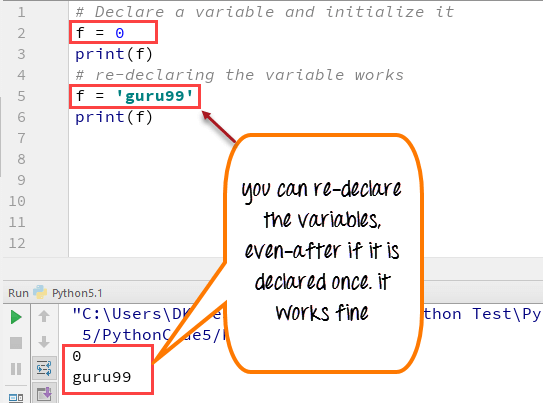Python 2 Example

``````# Declare a variable and initialize it
f = 0
print f
# re-declaring the variable works
f = 'guru99'
print f``````

Python 3 Example

``````# Declare a variable and initialize it
f = 0
print(f)
# re-declaring the variable works
f = 'guru99'
print(f)``````

## Python String Concatenation and Variable

Let’s see whether you can concatenate different data types like string and number together. For example, we will concatenate “Guru” with the number “99”.

Unlike Java, which concatenates number with string without declaring number as string, while declaring variables in Python requires declaring the number as string otherwise it will show a TypeError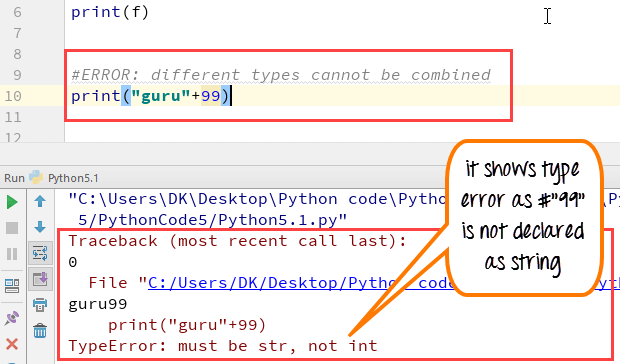For the following code, you will get undefined output –

``````a="Guru"
b = 99
print a+b``````

Once the integer is declared as string, it can concatenate both “Guru” + str(“99”)= “Guru99” in the output.

``````a="Guru"
b = 99
print(a+str(b))``````

## Python Variable Types: Local & Global

There are two types of variables in Python, Global variable and Local variable. When you want to use the same variable for rest of your program or module you declare it as a global variable, while if you want to use the variable in a specific function or method, you use a local variable while Python variable declaration.

Let’s understand this Python variable types with the difference between local and global variables in the below program.

1. Let us define variable in Python where the variable “f” is global in scope and is assigned value 101 which is printed in output
2. Variable f is again declared in function and assumes local scope. It is assigned value “I am learning Python.” which is printed out as an output. This Python declare variable is different from the global variable “f” defined earlier
3. Once the function call is over, the local variable f is destroyed. At line 12, when we again, print the value of “f” is it displays the value of global variable f=101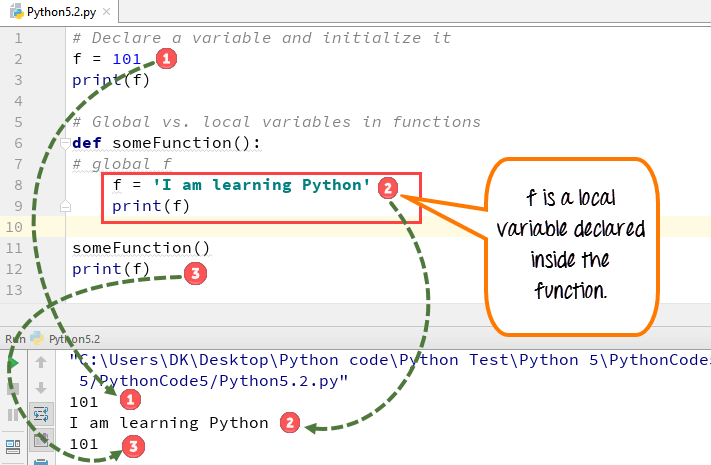Python 2 Example

``````# Declare a variable and initialize it
f = 101
print f
# Global vs. local variables in functions
def someFunction():
# global f
f = 'I am learning Python'
print f
someFunction()
print f``````

Python 3 Example

``````# Declare a variable and initialize it
f = 101
print(f)
# Global vs. local variables in functions
def someFunction():
# global f
f = 'I am learning Python'
print(f)
someFunction()
print(f)``````

While Python variable declaration using the keyword global, you can reference the global variable inside a function.

1. Variable “f” is global in scope and is assigned value 101 which is printed in output
2. Variable f is declared using the keyword global. This is NOT a local variable, but the same global variable declared earlier. Hence when we print its value, the output is 101

We changed the value of “f” inside the function. Once the function call is over, the changed value of the variable “f” persists. At line 12, when we again, print the value of “f” is it displays the value “changing global variable”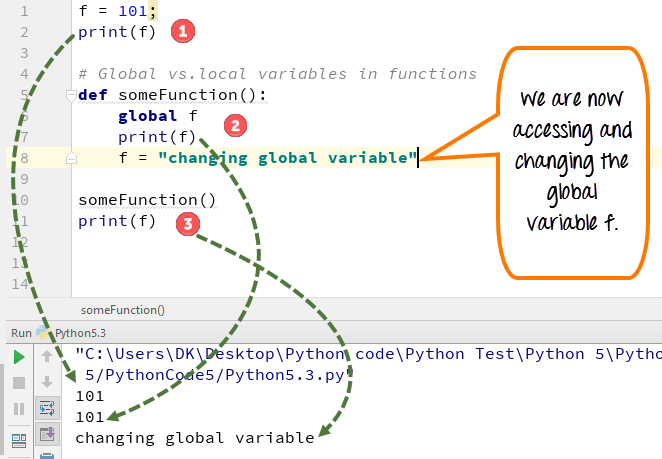Python 2 Example

``````f = 101;
print f
# Global vs.local variables in functions
def someFunction():
global f
print f
f = "changing global variable"
someFunction()
print f``````

Python 3 Example

``````f = 101;
print(f)
# Global vs.local variables in functions
def someFunction():
global f
print(f)
f = "changing global variable"
someFunction()
print(f)``````

## Delete a variable

You can also delete Python variables using the command del “variable name”.

In the below example of Python delete variable, we deleted variable f, and when we proceed to print it, we get error “variable name is not defined” which means you have deleted the variable.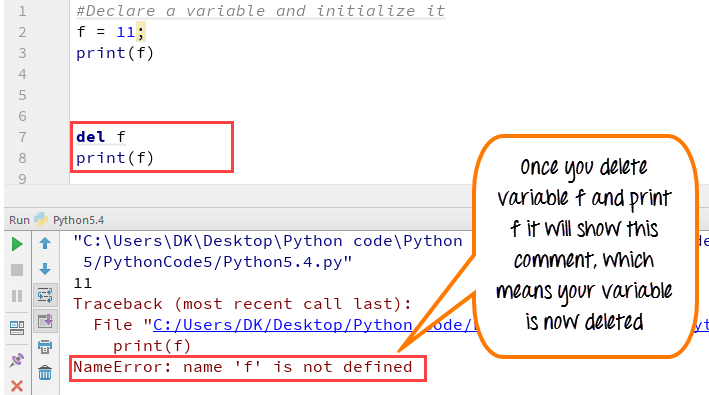Example of Python delete variable or Python clear variable :

``````f = 11;
print(f)
del f
print(f)``````

## Summary:

• Variables are referred to “envelop” or “buckets” where information can be maintained and referenced. Like any other programming language Python also uses a variable to store the information.
• Variables can be declared by any name or even alphabets like a, aa, abc, etc.
• Variables can be re-declared even after you have declared them for once
• Python constants can be understood as types of variables that hold the value which can not be changed. Usually Python constants are referenced from other files. Python define constant is declared in a new or separate file which contains functions, modules, etc.
• Types of variables in Python or Python variable types : Local & Global
• Declare local variable when you want to use it for current function
• Declare Global variable when you want to use the same variable for rest of the program

To delete a variable, it uses keyword “del”.

## A Beginner’s Guide To Python Variables

A variable is a fundamental concept in any programming language. It is a reserved memory location that stores and manipulates data. This tutorial on Python variables will help you learn more about what they are, the different data types of variables, the rules for naming variables in Python. You will also perform some basic operations on numbers and strings. We’ll use Jupyter Notebook to implement the Python codes.

Variables are entities of a program that holds a value. Here is an example of a variable:

x=100

In the below diagram, the box holds a value of 100 and is named as x. Therefore, the variable is x, and the data it holds is the value.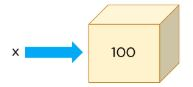The data type for a variable is the type of data it holds.

In the above example, x is holding 100, which is a number, and the data type of x is a number.

In Python, there are three types of numbers: Integer, Float, and Complex.

Integers are numbers without decimal points. Floats are numbers with decimal points. Complex numbers have real parts and imaginary parts.

Another data type that is very different from a number is called a string, which is a collection of characters.

Let’s see a variable with an integer data type:

``x=100``

To check the data type of x, use the type() function:

``type(x)``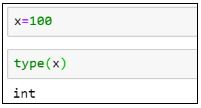Python allows you to assign variables while performing arithmetic operations.

``````x=654*6734
type(x)``````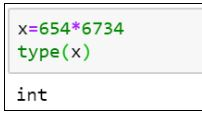To display the output of the variable, use the print() function.

``print(x) #It gives the product of the two numbers``

Now, let’s see an example of a floating-point number:

``````x=3.14
print(x)``````

type(x) #Here the type the variable is float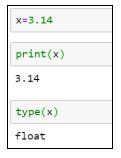Strings are declared within a single or double quote.

``````x=’Simplilearn’

print(x)

x=” Simplilearn.”

print(x)

type(x)``````

In all of the examples above, we only assigned a single value to the variables. Python has specific data types or objects that hold a collection of values, too. A Python List is one such example.

Here is an example of a list:

``````x=[14,67,9]

print(x)

type(x)``````

You can extract the values from the list using the index position method. In lists, the first element index position starts at zero, the second element at one, the third element at two, and so on.

To extract the first element from the list x:

``print(x)``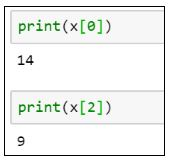To extract the third element from the list x:

``print(x)``

Lists are mutable objects, which means you can change the values in a list once they are declared.

``````x=70 #Reassigning the third element in the list to 70

print(x)``````

Earlier, the elements in the list had [14, 67, 9]. Now, they have [14, 67, 70].

Tuples are a type of Python object that holds a collection of value, which is ordered and immutable. Unlike a list that uses a square bracket, tuples use parentheses.

``````x=(4,8,6)

print(x)

type(x)``````

Similar to lists, tuples can also be extracted with the index position method.

``print(x) #Give the element present at index 1, i.e. 8``

If you want to change any value in a tuple, it will throw an error. Once you have stored the values in a variable for a tuple, it remains the same.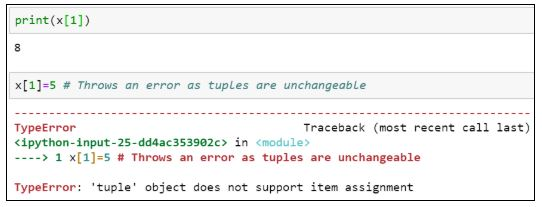When we deal with files, we need a variable that points to it, called file pointers. The advantage of having file pointers is that when you need to perform various operations on a file, instead of providing the file’s entire path location or name every time, you can assign it to a particular variable and use that instead.

Here is how you can assign a variable to a file:

``````x=open(‘C:/Users/Simplilearn/Downloads/JupyterNotebook.ipynb’,’r’)

type(x)``````

Suppose you want to assign values to multiple variables. Instead of having multiple lines of code for each variable, you can assign it in a single line of code.

``(x, y, z)=5, 10, 5``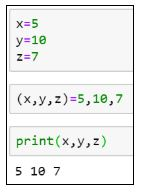The following line code results in an error because the number of values assigned doesn’t match with the number of variables declared.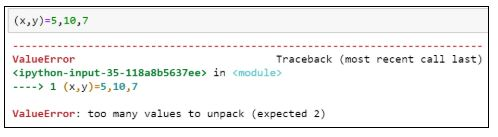If you want to assign the same value to multiple variables, use the following syntax:

``x=y=z=1``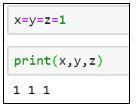Now, let's look at the various rules for naming a variable.

1. A variable name must begin with a letter of the alphabet or an underscore(_)

Example:

``````abc=100 #valid syntax

_abc=100 #valid syntax

3a=10 #invalid syntax

@abc=10 #invalid syntax``````

. The first character can be followed by letters, numbers or underscores.

Example:

``````a100=100 #valid

_a984_=100 #valid

a9967\$=100 #invalid

xyz-2=100 #invalid``````

Python variable names are case sensitive.

Example:

``````a100 is different from A100.

a100=100

A100=200``````

Reserved words cannot be used as variable names.

Example:

``````break, class, try, continue, while, if

break=10

class=5

try=100``````

Python is more effective and more comfortable to perform when you use arithmetic operations.

The following is an example of adding the values of two variables and storing them in a third variable:

``````x=20

y=10

result=x+y

print(result)``````

Similarly, we can perform subtraction as well.

``````result=x-y

print(result)``````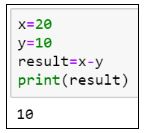Additionally, to perform multiplication and division, try the following lines of code:

``````result=x*y

print(result)

result=x/y

print(result)``````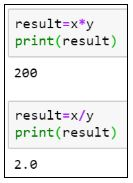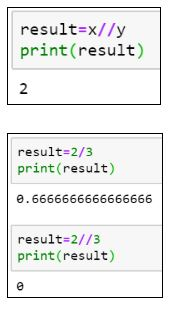As you can see, in the case of division, the result is not an integer, but a float value. To get the result of the division in integers, use “//”the integer division.

The division of two numbers gives you the quotient. To get the remainder, use the modulo (%) operator.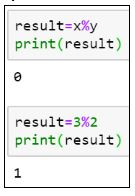Now that we know how to perform arithmetic operations on numbers let us look at some operations that can be performed on string variables.

``var = ‘Simplilearn’``

You can extract each character from the variable using the index position. Similar to lists and tuples, the first element position starts at index zero, the second element index at one, and so on.

``````print(var) #Gives the character at index 0, i.e. S

print(var) #Gives the character at index 4, i.e. l``````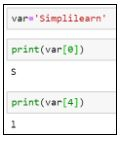If you want to extract a range of characters from the string variable, you can use a colon (:) and provide the range between the ones you want to receive values from. The last index is always excluded. Therefore, you should always provide one plus the number of characters you want to fetch.

``print(var[0:3]) #This will extract the first three characters from zero, first, and second index.``

The same operation can be performed by excluding the starting index.

``print(var[:3])``The following example prints the values from the fifth location until the end of the string.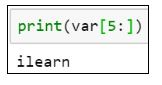Let’s see what happens when you try to print the following:

``print(var[0:20]) #Prints the entire string, although the string does not have 20 characters.``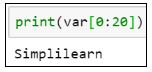To print the length of a string, use the len() function.

``len(var)``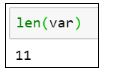Let’s see how you can extract characters from two strings and generate a new string.

``````var1 = “It’s Sunday”

var2 = “Have a great day”``````

The new string should say, “It’s a great Sunday” and be stored in var3.

``````var3 = var1[:5] + var2[5:13] + var1[5:]

print(var3)``````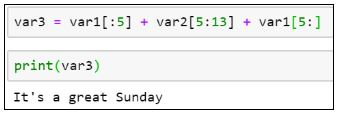Get prepared for your next career as a professional Python programmer with the Python Certification Training Course. Click to enroll now!

## Conclusion

I hope this blog helped you learn the concepts of Python variables. After reading this blog, you may have learned more about what a variable is, rules for declaring a variable, how to perform arithmetic operations on variables, and how to extract elements from numeric and string variables using the index position.

#python #programming1620737688

## Top Cross-Platform App Developers in USA

Are you looking for the best Cross-Platform app developers in USA? AppClues Infotech has the best expertise to create mobile app on Android & iOS platform. Get in touch with our team for the right Cross-Platform app development solution.

Website: https://www.appcluesinfotech.com/
Email: info@appcluesinfotech.com
Call: +1-978-309-9910

#cross-platform app development #cross-platform framework development #cross-platform mobile app development #top cross platform app developers in usa #top cross platform app developers in usa #develop a cross-platform mobile app1623323207

## Platform App Design | Cross-Platform Development Services

Cross-Platform Development Services

With the development in mobile app technology, a huge time saver as well as the quality maintainer technology is Cross-Platform App development. The development of an app that takes less time to develop as well as uses one technology to develop an app for both android and iOS is game-changing technology in mobile app development.

Want to develop or design a Cross-platform app?

With the successful delivery of more than 950 projects, WebClues Infotech has got the expertise as well as a huge experience of cross-platform app development and design. With global offices in 4 continents and a customer presence in most developed countries, WebClues Infotech has got a huge network around the world.

Want to know more about our cross-platform app designs?

View Portfolio https://www.webcluesinfotech.com/portfolio/

#cross-platform development services #cross platform mobile app development services #cross-platform mobile app development services #cross platform app development services #hire cross platform app developer #hire cross-platform app developer india usa,1616583098

## Cross Platform Mobile App Development Company in USA

AppClues Infotech is a top-notch cross platform mobile app development company in USA. With strong mobile app designers & developers team that help to create powerful cross-platform apps using the current market technologies & functionalities.